## Ising Model

The Ising model considers a lattice of paricles that have up or down spin. The total energy is given by the interaction of nearest neighbors and interaction of the spin with an external field.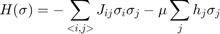Where the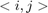means adjacent particles,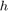is the field,is the spin, which can be either -1 or 1, and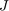is the interaction energy.

https://en.wikipedia.org/wiki/Ising_model

## The 1D Ising Model

clc
close all
clear all

Beta=1;         %1/KbT
J=0;            %Interaction Strength
B=2;            %Magnetic Field
N=40;           %Number of spins

Spins=double(randn(N,1)>0);
Spins(Spins==0)=-1;

%Plot Spins
X=(1:N)';
Y=Spins==-1;
FigH=figure;
FigH.Position=[200,300,900,400];
quiver(X,Y,X*0,Spins,0,'linewidth',2)
axis([0 N+1 -1 2])
s=sprintf('Beta=%g, J=%g, B=%g',Beta,J,B);
title(s)

%Setup so we stop when figure is closed
FigH.CloseRequestFcn='Go=0;';
Go=1;

%Run Markov Chain Monte Carlo
NChain=1000000;
nn=0;
while Go
nn=nn+1;    %counter for display

%Make test jump

%Pick site and flip spin
ID=ceil(N*rand);
SpinsTest=Spins;
SpinsTest(ID)=-Spins(ID);

%Energy from nearest neighbor interactions
E_Spin=J*(Spins(1:end-1).*Spins(2:end)+Spins(1)*Spins(end));
E_SpinTest=J*(SpinsTest(1:end-1).*SpinsTest(2:end)+SpinsTest(1)*SpinsTest(end));

%Energy from Interactions with feild
E_Field=-B*sum(Spins);
E_FieldTest=-B*sum(SpinsTest);

%Total Energy (Hamiltonian)
H=E_Spin+E_Field;
HTest=E_SpinTest+E_FieldTest;

%Test acceptance probability for Metropolis-Hastings
Alpha=exp(-Beta*(HTest-H));

%Metropolis-Hastings rule
if rand()< (Alpha)
Spins(ID)=-Spins(ID);
end

ShowIter=1;
%Show every ShowIter interations
if nn/ShowIter==round(nn/ShowIter)
figure(FigH);
hold off
Y=Spins==-1;
quiver(X,Y,X*0,Spins,0,'linewidth',2)
axis([-1 N+1 -3 4])
title(s)
hold on
quiver(0,0,0,B,0,'linewidth',2)
legend('Spins','Field')
pause(.1)
end
end
delete(FigH)
figure;
hold off
Y=Spins==-1;
quiver(X,Y,X*0,Spins,0,'linewidth',2)
axis([-1 N+1 -3 4])
title(s)
hold on
quiver(0,0,0,B,0,'linewidth',2)
legend('Spins','Field')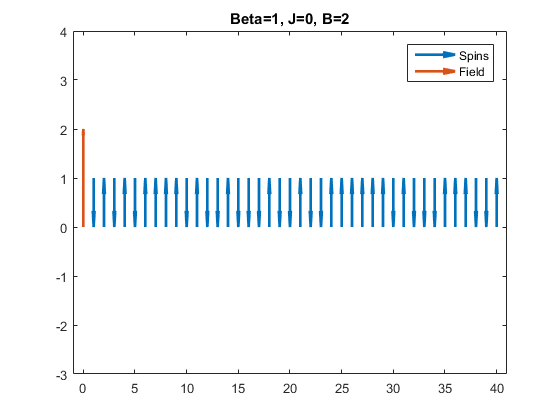## 2D Ising Model

Same rules as the 1D Ising model. Neighbors are the 4 closest spins. In 2D there is phase transition at -1/(Beta*J)=2/log(1+sqrt(2))

clc
close all
clear all

Beta=10;         %1/KbT
J=-.2;            %Interaction Strength
B=0;            %Magnetic Field
N=100;           %Number of spins

2/log(1+sqrt(2))
-1/(Beta*J)

Spins=double(randn(N,N)>0);
Spins(Spins==0)=-1;

%Plot Spins
FigH=figure;
%FigH.Position=[200,200,900,400];
imshow(Spins,[],'InitialMagnification',400)
s=sprintf('Beta=%g, J=%g, B=%g',Beta,J,B);
title(s)

%Setup so we stop when figure is closed
FigH.CloseRequestFcn='Go=0;delete(FigH)';
Go=1;

%Run Markov Chain Monte Carlo
NChain=1000000;
nn=0;
while Go
nn=nn+1;    %counter for display

%Make test jump

%Pick site and flip spin
IDX=ceil(N*rand);
IDY=ceil(N*rand);
SpinsTest=Spins;
SpinsTest(IDX,IDY)=-Spins(IDX,IDY);

%Energy from nearest neighbor interactions
E_Spin=J*(sum(sum(Spins(1:end-1,:).*Spins(2:end,:)))+... %Y neighbor
sum(Spins(1,:).*Spins(end,:))+... %Y periodic BC
sum(sum(Spins(:,1:end-1).*Spins(:,2:end)))+... %X neighbor
sum(Spins(:,1).*Spins(:,end))); %X periodic BC

E_SpinTest=J*(sum(sum(SpinsTest(1:end-1,:).*SpinsTest(2:end,:)))+... %Y neighbor
sum(SpinsTest(1,:).*SpinsTest(end,:))+... %Y periodic BC
sum(sum(SpinsTest(:,1:end-1).*SpinsTest(:,2:end)))+... %X neighbor
sum(SpinsTest(:,1).*SpinsTest(:,end))); %X periodic BC

%Energy from Interactions with feild
E_Field=-B*sum(Spins(:));
E_FieldTest=-B*sum(SpinsTest(:));

%Total Energy (Hamiltonian)
H=E_Spin+E_Field;
HTest=E_SpinTest+E_FieldTest;

%Test acceptance probability for Metropolis-Hastings
Alpha=exp(-Beta*(HTest-H));

%Metropolis-Hastings rule
if rand()< (Alpha)
Spins(IDX,IDY)=-Spins(IDX,IDY);
end

ShowIter=1000;
%Show every ShowIter interations
if (nn/ShowIter==round(nn/ShowIter))
pause(.01);if ~Go;break;end % Time to process closed figure
figure(FigH);
try
imshow(Spins,[],'InitialMagnification',400)
catch
end
pause(.01)
end
end

ans =

2.2692

ans =

0.5000